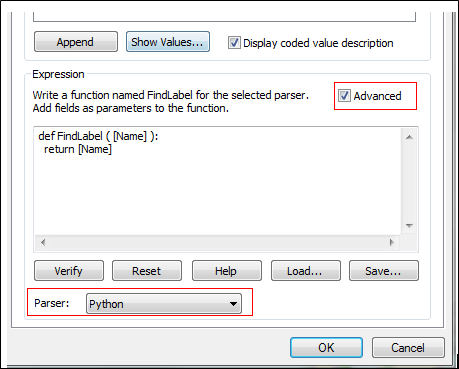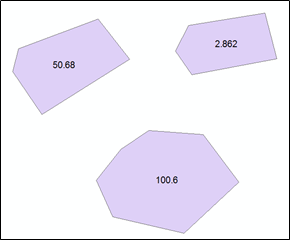## 10 Python ArcGIS Label Expression Code

Python scripting has become popular with the ArcGIS software. ArcGIS adopted it after version 9.0. Python script can be used in many parts within ArcGIS; label expression, attribute calculator, model builder or geoprocessing tools. Label expression provides option for Python, VB Script and Jscript. On this tutorial I have written 10 python script that can be used in your daily GIS work. This scenario are something you might encounter that cannot be handled by Maplex extension.

To insert the code, Open the Layer Properties > Labels > ExpressionsThen select the python from the parser and check the Advanced box1. TEXT Format: This example demonstrate how to convert your text strings to different format.

A. Convert text string to upper or lower case

def FindLabel ( [Name] ):
L = [Name]
L = L. lower()
return

Note:    For upper case use function upper()
For proper case use function proper()

B. Stacking of the fields, for example if you want three fields to be displayed in the stack. For this you do not need to check Advance box, so uncheck it.

Example:             City Name:  Calgary
1000
NE

“City Name: ” + [NAME] + ‘\n’ + [Number] + ‘\n’ + [Direction]

2. Stack Field: Next example is to stack the text from the single field. Again check the Advance box if you have unchecked it. On the below example attribute values has the comma and stacking will be happing based on it.

Name field: Calgary, 1000, NE will label as

City Name:         Calgary
1000
NE

def FindLabel ( [NAME]  ):
L = [NAME]
L = L.replace(‘, ‘, ‘\n’)
return L

3.  Color and Format Label: If you want to put some condition on the font; if there value meet some condition then change the color and size. Below example will change the font color to blue and size will be bold if there population is more than 5000 and others will be default value.

def FindLabel ( [NAME], [POPULATION] ):
if long([POPULATION]) >= 5000:
return “<CLR red=’255′><FNT size = ’14’>” + [NAME] + “</FNT></CLR>”
else:
return [NAME]

4. Split Text: Let us say you have Name field that has the value “ Calgary,100” and you want to plot value after “,“ that is 100 , then use the python function called split ().

def FindLabel ( [Name] ):
return [Name].split(“,”)

5. Exclude, Concatenate and Stack: You have Name field whose value is “ BP10200” and next field Code that has value “546”. You want to plot both the fields but excluding number from first field and your result will be:

BP
546

def FindLabel ( [Name] , [Code]   ):
return [Name] [:2] + ‘\n’ + [Code]

6. Replace command: If you have field name with long string and you want to replace with short form then use function called “replace”. For example your name field has “CountyRoads2340” and you want to label as “CR2340”.

Results: CR2340

def FindLabel ( [Name]):

7. Removing non-numeric characters: Sometime you want to plot only numbers from the alphanumeric. For example if we have text like “ 2 Highway, 22 minor road, 74 street” and you want to plot only 2 22 74 .

def FindLabel ([Name]):
import re
output = re.sub(“[^0-9 ]”, “”, [Name])
return output

8. Concatenation with Condition: Let us say you have two fields and you want to plot both, then you can concatenate it using simple expression: [Name] + ” ” + [Code]. But scenario might be different, if one field is null and you still want to plot other field.

def FindLabel([Name],[Code]):
if str([Name]) == “None” and str([Code]) != “None”:
return [Code]
elif str([Name]) != “None” and str([Code]) == “None”:
return [Name]
elif str([Name]) == “None” and str([Code]) == “None”:
return “”
else:
return str([Name]) + ” ” + str([Code])

9. Count values: If values are repeating on the field and you want to count it; for example A(2) which means A is repeating 2 times. Let us say you have field name called “name” which have two “A” then labeling will display as A(2)

Note: on below code Name is the field name and Example is the layer name.

def FindLabel ( [Name] ):
mxd = arcpy.mapping.MapDocument(“CURRENT”)
lyr = arcpy.mapping.ListLayers (mxd,”Example”)
q='”NAME”=’+”‘”+[NAME]+”‘”
tbl=arcpy.da.TableToNumPyArray(lyr,”Name”,q)
n=len(tbl)
return ‘%s(%s)’ %([Name],str(n))

10. Rounding Values: To label numeric fields and want to round off based on the condition. For example if value is more than 1 and less 50 then 3 decimal, if between 50 to 100  then 2 decimal and others 1 decimal.def FindLabel([Code]):
if [Code] is not None:
value = float([Code])
if 1 < value < 50:
return round(value, 3)
elif 50 <= value < 100:
return round(value, 2)
else:
return round(value, 1)
else:
return NoneThank you for reading my tutorial, if you have any question please post on the comment box. If you want to take free course about the python, please visit Free Python Course.

### This Post Has One Comment

1.def FindLabel ( [NAME] ):
L = [“Tacoma”, “Seattle”, “Olympia”, “Bellevue”, “Everett”]
for i in L:
if i in [NAME]:
return [NAME]
else:
pass

This site uses Akismet to reduce spam. Learn how your comment data is processed.